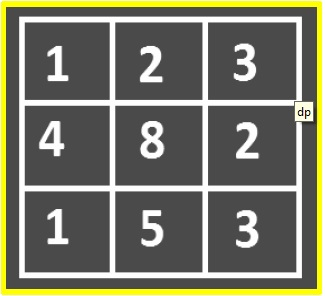# C Program for Minimum Cost Path

Here, we will solve the minimum cost path problem in C. The implication is done on a 2D-matrix where each cell has a cost to travel. We have to find a path from the left top corner to the bottom right corner with minimum travel cost. You can only traverse down and right lower cells from a given cell.

To solve this particular problem dynamic programming is much better to approach than recursion.

Given cost matrix cost[ ][ ] and a position (m,n), we have to write a function that returns cost of minimum path to reach (m,n) from (0,0).The total cost of a path to reach (m, n) is the sum of all the costs on that path (including both source and destination).

Assumption − All costs are positive. Negative cost cycles do not exist in the input matrix

## Example

Find the minimum cost path to (2,2)The costs are given within the image itself. The path will be (0, 0) ⇒ (0, 1) ⇒ (1, 2) ⇒ (2, 2). The value of the path is eight (1 +2+2+ 3).

Approach − Create an answer matrix of a similar size as the given matrix.

Fill this matrix in a bottom-up manner.

Given − arrA[ ][ ]. At each cell, we’ve got 2 options(go right or down) and that we can select the minimum of those 2, for any i,j cell.

solution[i][j]=A[j] if i=0, 1st row
=A[i] if j=0, 1st column
=A[i][j]+Min(solution[i=1],[j],solution[i][j-1]) if i>0 && j>0

The approach followed in the algorithmic answers can be used to efficiently solve this problem by applying dynamic programming. Create a minimum cost path table of size m,n and define−

minimumCostPath[i][j] = minimum value to achieve (i, j) from (0, 0)

Clearly,

minimumCostPath = costMatrix
minimumCostPath[i] = minimumCostPath[i - 1] + costMatrix[i], for all values of i > zero
minimumCostPath[j] = minimumCostPath[j - 1] + costMatrix[j], for all values of j >zero

Next, we will fill the minimum cost path matrix by applying a similar formula that we applied in the algorithm. Since all the previous values will already have been calculated within the minimum cost path matrix, we will not recalculate these as we did in the algorithmic answer.

minimumCostPath[i][j] = costMatrix[i][j] +minimum(minimumCostPath[i - 1][j - 1],minimumCostPath[i - 1][j],minimumCostPath[i][j - 1])

Here, for calculating minimumCostPath[i][j] we tend to use minimumCostPath[i - 1][j - 1], minimumCostPath[i - 1][j] and minimumCostPath[i][j - 1] as a result, these are the sole permissible cells from which we will reach minimumCostPath[i][j].Finally, we return minimumCostPath[m][n].

The time complexity of the dynamic programming algorithm is O(mn).

## Example

Live Demo

#include <iostream>
using namespace std;
int min_(int a, int b, int c){
if (a < b)
return (a < c) ? a : c;
else
return (b < c) ? b : c;
}
int min_cost(int cost, int m, int n){
int i, j;
int tot_cost;
tot_cost = cost;
for (i = 1; i <= m; i++)
tot_cost[i] = tot_cost[i - 1] + cost[i];
for (j = 1; j <= n; j++)
tot_cost[j] = tot_cost[j - 1] + cost[j];
for (i = 1; i <= m; i++)
for (j = 1; j <= n; j++)
tot_cost[i][j] = min_(tot_cost[i - 1][j - 1], tot_cost[i - 1][j], tot_cost[i][j - 1]) + cost[i][j];
}
int main(){
int cost = {
{ 9, 9, 4 },
{ 8, 0, 9 },
{1, 2, 8}
};
cout<<" The minimum cost is "<<min_cost(cost, 2, 2);
return 0;
}

## Output

The minimum cost is 17